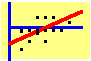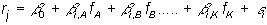8.4 Multi-Index Models

The multi-index model assumes a return-generating process that is a linear function of many factors. In this approach, each factor is a source of systematic risk. Since investors cannot diversify systematic risk, they are assumed to be compensated for bearing this risk.

As a result, a security's sensitivity to each factor affects the assumed return-generating process for the security. This sensitivity is captured by a "factor beta," and the return-generating process is assumed to take the formThe last term is the source of idiosyncratic or diversifiable risk. Except for the fact that multiple factors make this a richer model than the single index model, the use of a multi-factor model is similar to the single-index model.

A broad generalization of these models is the Arbitrage Pricing Theory.

previous topic

next topic